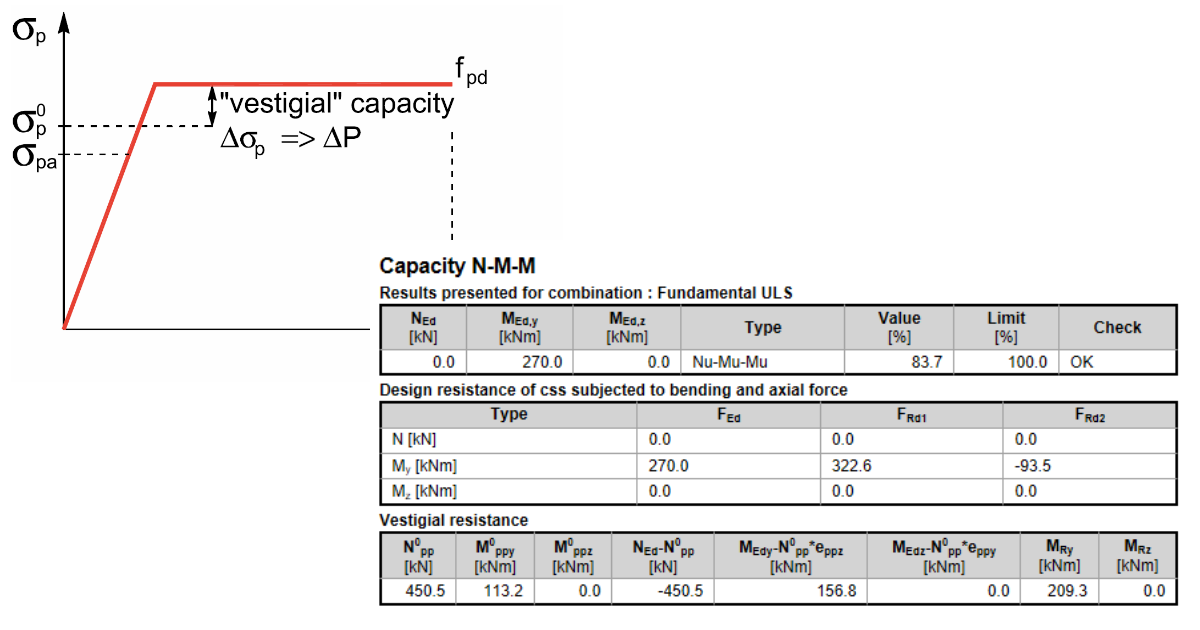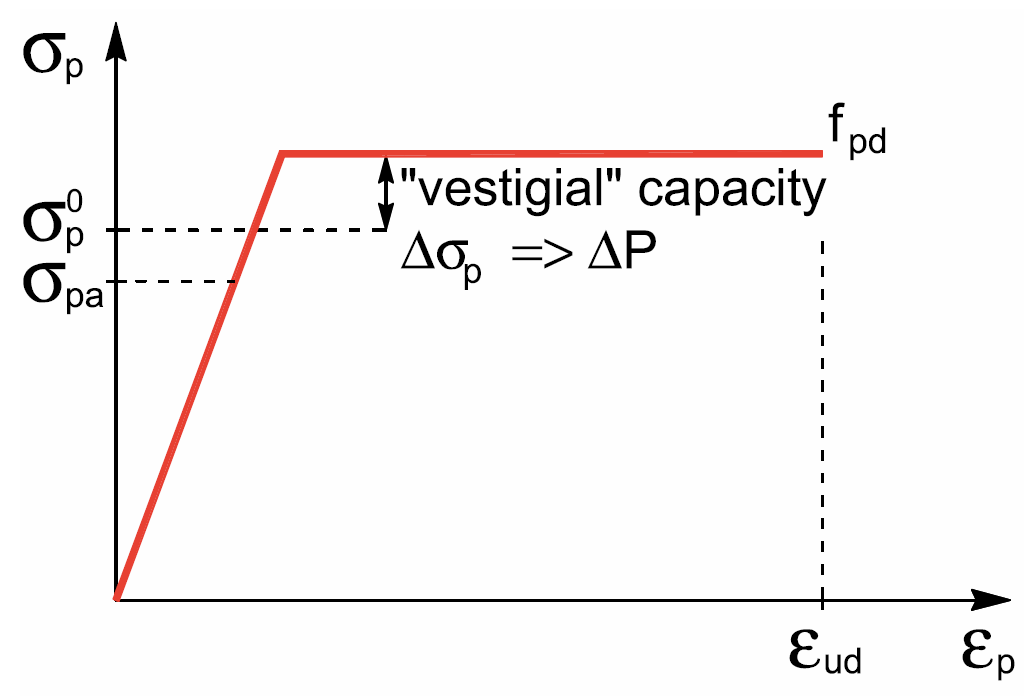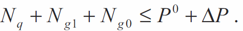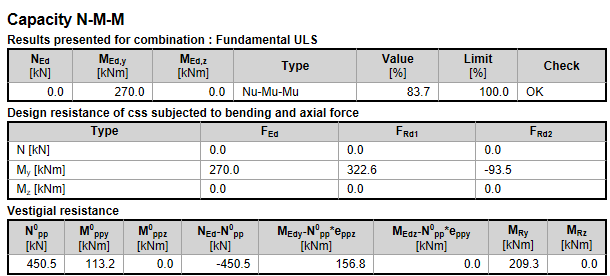### Choose language# Vestigial resistance

$$The most frequent usage of vestigial resistance is for prestressed members where is important to know the reserve to maximal capacity of the subjected member for the combination N+M+M.You can use the following inequation for axial force.The left-hand side of the inequality contains the external load and the right-hand side of the resistance. Generally, the right-hand side then contains the primary effects resulting from the decompression prestressing force and the effects of the vestigial resistance of the prestressing reinforcement.As a consequence of this, you cannot find prestressing force P on the side of outer loads in IDEA StatiCa. It' being seen in the following tab.N0pp stands for primary force causing decompression stress in prestressed reinforcementNEd stands for external load – in this case, the external load was equal to zero$$